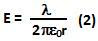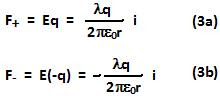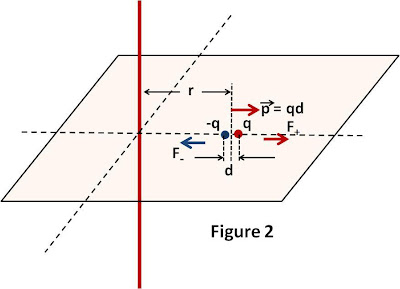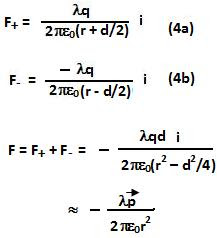## Friday, July 2, 2010

### Irodov Problem 3.46

a)The dipole moment p can be represented as two charges q and -q separated by a distance d along the z=axis, located at a distance r from the wire as shown in Figure 1. ThenWe already know from 3.22 Eqn 1, that the electric field due to a long wire at a distance r from its axis is given by,The force on positive and negative electric charges in the dipole are then respectively given by,The net force on the dipole is then given by,b)Similar to part a, now the charges are located along the x-axis. The force is then given by,c)Similar to parts a and b, the charges are located along the y-axis. The force is then given by,